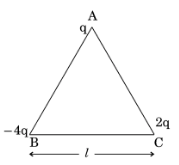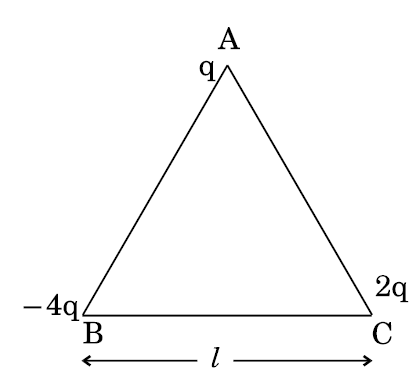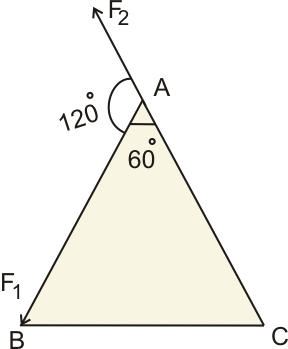# (a) Three point charges q, – 4q and 2q are placed at the vertices of an equilateral triangle ABC of side ‘l’ as shown in the figure. Obtain the expression for the magnitude of the resultant electric force acting on the charge q.(b) Find out the amount of the work done to separate the charges at infinite distanceBy coulomb's law force between two charges is

where q1 and q2 are charges separated by distance r
Here, to find the force on the charge at A by charge at B and C
Force acting on charge q by -4q is

Force acting on charge q by 2q isFor the above diagram, the resultant force acting on charge at
A is,

here,    and

So-net  force on q is

,
b) The amount of the work done to separate the charges at infinite distance is equal to the potential energy of the system.
The potential energy between two charges separated by a distance 'r' is

Here the potential energy of the system is,

therefore,

work done is

## Related Chapters

### Preparation Products

##### Knockout NEET Sept 2020

An exhaustive E-learning program for the complete preparation of NEET..

₹ 15999/- ₹ 6999/-
##### Rank Booster NEET 2020

This course will help student to be better prepared and study in the right direction for NEET..

₹ 9999/- ₹ 4999/-
##### Knockout JEE Main Sept 2020

An exhaustive E-learning program for the complete preparation of JEE Main..

₹ 12999/- ₹ 6999/-
##### Test Series NEET Sept 2020

Take chapter-wise, subject-wise and Complete syllabus mock tests and get in depth analysis of your test..

₹ 4999/- ₹ 2999/-